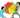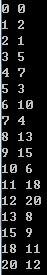## 自然数大分家

Wed, 27th November 2019Edit on Githubsequence

# 自然数分家 - 威佐夫博弈和Beatty定理

PKU-1067是一个石子游戏，这其实是一个威佐夫博弈问题, 英文维基百科 也有详尽描述。$b_k=a_k+k\gt a_{k−1}+k\gt a_{k−1}+k−1=b_{k−1}\gt a_{k−1}$

## Beatty定理

$a，b$是正无理数且$\frac1a+\frac1b=1$
$P=\{\lfloor na\rfloor|n为任意的正整数\}，Q=\{\lfloor nb\rfloor|n为任意的正整数\}$
$\lfloor x\rfloor$指的是不好过$x$的最大整数）
$P$$Q$$Z^{+}$的一个划分，即$P∩Q$为空集且$P∪Q$为正整数集合$Z^{+}$

# 三家分赵

{a(n)}={1, 4, 07, 09, 12, 15, 16, 19, 22, 25, 27, 30, 32, 34, 37, 40, 43, 45, 47, 50, 52, 55, 58, 60, 63, 65,...}
{b(n)}={2, 5, 08, 11, 14, 18, 20, 23, 26, 29, 33, 36, 38, 41, 44, 48, 51, 54, 56, 59, 62, 66, 69, 72, 75, 77,...}
{c(n)}={3, 6, 10, 13, 17, 21, 24, 28, 31, 35, 39, 42, 46, 49, 53, 57, 61, 64, 67, 71, 74, 79, 82, 85, 89, 92,...}

$a(n)=n+\lfloor n\sqrt{\frac12}\rfloor+\lfloor n\sqrt{\frac14}\rfloor$

$b(n)=n+\lfloor n\sqrt{\frac12}\rfloor+\lfloor n\sqrt{2}\rfloor$

$c(n)=n+\lfloor n\sqrt{4}\rfloor+\lfloor n\sqrt{2}\rfloor$

lsr314分析发现 , 貌似只要$t\gt 0$$t^2,t+\frac1t,t+t^2,\frac1t+\frac1{t^2}$都是无理数，那么 $a_n=n+\lfloor\frac nt\rfloor+\lfloor\frac n{t^2}\rfloor,b_n=n+\lfloor\frac nt\rfloor+\lfloor nt\rfloor,c_n=n+\lfloor\frac n{t^2}\rfloor+\lfloor nt\rfloor,$ $\lfloor x\rfloor$表示$x$的整数部分，那么每个正整数刚好在$a_n,b_n,c_n$中出现一次。本题中$t=2^{\frac14}$

# 群雄逐鹿

lsr314继续做出解答

$a_i(n)=\sum_{j=1}^k\lfloor\frac{t_j}{t_i} n\rfloor,i=1,2,…k.$ 那么每个正整数在数列$a_1(n),…a_k(n)$中恰好出现一次。

# 自然数大分家 - Fibonacci矩阵

mathe在csdn的博客 中, 如下定义一个无限阶矩阵m：

$\begin{matrix} 1&2&3&5&8&13&21&34&55&89&144&\dots\\ 4&7&11&18&29&47&76&123&199&322&521&\dots\\ 6&10&16&26&42&68&110&178&288&466&754&\dots\\ 9&15&24&39&63&102&165&267&432&699&1131&\dots\\ 12&20&32&52&84&136&220&356&576&932&1508&\dots\\ 14&23&37&60&97&157&254&411&665&1076&1741&\dots\\ \vdots&\vdots&\vdots&\vdots&\vdots&\vdots&\vdots&\vdots&\vdots&\vdots&\vdots&\ddots \end{matrix}$

32332...

32

32332

3233232

3233232332

Github